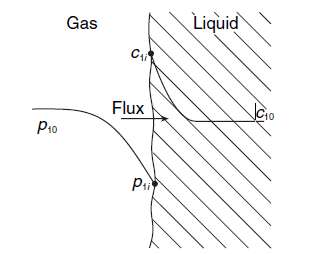# Derivation of the Overall Mass Transfer Coefficient

## Homework StatementThe problem requires me to find the overall mass transfer coefficient or Kg.

## Homework Equations

$$N_{1}=\frac{1}{\frac{1}{k_{p}+\frac{H}{k_{x}}}}(p_{1}-Hx_{1}), (1)$$
$$N_{1}=k_{p}(p_{1}-p_{1i}), (2)$$
$$N_{1}=k_{x}(x_{1i}-x_{1}), (3)$$

## The Attempt at a Solution

So, I need to use equations 2 and 3 to get equation 1. The driving force:
$$(p_{1}-Hx_{1})$$
comes from using Henry's law, $$P_{1}=Hx_{1}$$ but I just do not understand where the overall mass transfer coefficient comes from, I have tried rearranging 2 and 3 to no avail, can someone help please.

Chestermiller
Mentor
Along with the equation ##p_{1i}=Hx_{1i}##, you have 3 linear algebraic equation in the 3 unknowns ##N_1##, ##p_{1i}## and ##x_{1i}##. Just solve these equations for ##N_1##.

•Tom Hardy
Along with the equation ##p_{1i}=Hx_{1i}##, you have 3 linear algebraic equation in the 3 unknowns ##N_1##, ##p_{1i}## and ##x_{1i}##. Just solve these equations for ##N_1##.

Thanks I did, I was just being silly before.